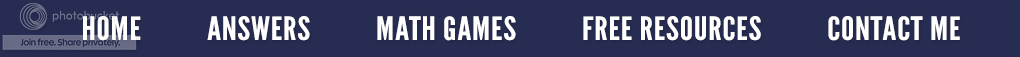### Is zero even? What an "odd" question!

My daughter and her husband are heading to Las Vegas with his family to celebrate his parent's 50th wedding anniversary.  I guess when you are at the roulette table, (never been there or done that) and you bet "even" and the little ball lands on 0 or 00, you lose. Yep, it's true; zero is not considered an even number on the roulette wheel, something you better know before you bet.  This example is a non-mathematical, real-life situation where zero is neither odd or even.  But in mathematics, by definition, zero is an even number. (An even number is any number that can be exactly divided by 2 with no remainder.)  In other words, an odd number leaves a remainder of 1 when divided by 2 whereas an even number has nothing left over.  Under this definition, zero is definitely an even number since 0 ÷ 2 = 0  has no remainder.

Zero also fits the pattern when you count which is the same as alternating even (E) and odd (O) numbers.
Most math books include zero as an even number; however, under special circumstances zero may be excluded.  (For example, when defining even numbers to mean even NATURAL numbers.)  Natural numbers are the set of counting numbers beginning with 1 {1, 2, 3, 4, 5....}; so, zero is not included.
Consider the following simple illustrations.  Let's put some numbers in groups of two and see what happens.

As you can see, even numbers such as 4 have no "odd man out" whereas odd numbers such as 3 always have one left over.  Similarly, when zero is split into two groups, there is not a single star that does not fit into one of the two groups.  Each group contains no stars or exactly the same amount.  Consequently, zero is even.

Algebraically, we can write even numbers as 2n where n is an integer while odd numbers are written as 2n + 1 where "n" is an integer.  If n = 0, then 2n = 2 x 0 = 0 (even) and 2n + 1 = 2 x 0 + 1 = 1 (odd).  All integers are either even or odd. (This is a theorem).  Zero is not odd because it cannot fit the form 2n + 1 where "n" is an integer. Therefore, since it is not odd, it must be even.

I know this seems much ado about nothing, but a great deal of discussion has surrounded this very fact on the college level.  Some instructors feel zero is neither odd or even.  (Yes, we like to debate things that seem obvious to others.)

Consider this multiple choice question.  (It might just appear on some important standardized test.)Which answer would you choose and why?

Zero is…
a.) even
b.) odd
c.) all of the above
d.) none of the above

Mathematically, I see zero as the count of no objects, or in more formal terms, it is the number of objects in the empty set.  Also, since zero is defined as an even number in most math textbooks, and is divisible by 2 with no remainder, then "a" is my answer.

### Odd Man Out!

Sometimes we think everyone knows the difference between an odd and even number.  When I was teaching my remedial math college class, we were learning the divisibility rules, the first of which is that every even number is divided by two.  I wrote the number "546" on the board and asked the class if this was an odd or even number.  I had one student who disagreed with the group answer of even.  I asked him why he thought the number was odd, and he replied, "Because it has a "5" in it. " It was obvious this student got all the way through high school without a clear understanding of odd and even numbers.  So the moral to this story is to be sure to discuss the difference between an even and an odd number with your students.
A good definition for an even number is that it can be put into groups of two without any left over, like giving each person a partner.  But when you have an odd number of things and put them into groups of two, one will always be left out.
Try this approach.  Make your hands into fists and place them side by side as seen in the illustration.  Say  a number.  Now count, and as you count, put up one finger for each number said, alternating between hands, with fingers touching.
For instance, if you said “4”, you would count one, (left pointer finger up) two, (right pointer finger up and touching the other pointer finger) three, (left middle finger up), four (right middle finger up, touching the other middle finger).  Four is even because each finger has a partner to touch.
Repeat this several times, giving the students odd as well as even numbers.  By always having a concrete visual (their fingers) will help the kinesthetic and visual learner to "see" the odds and evens.

Activities such as this can be found in a 24 page booklet entitled Number Tiles for The Primary Grades.   It contains 17 different math problem solving activities that extend from simple counting, to even and odd numbers, to greater than or less than to solving addition and subtraction problems.
OR.....Check out the ten page free version by clicking here...